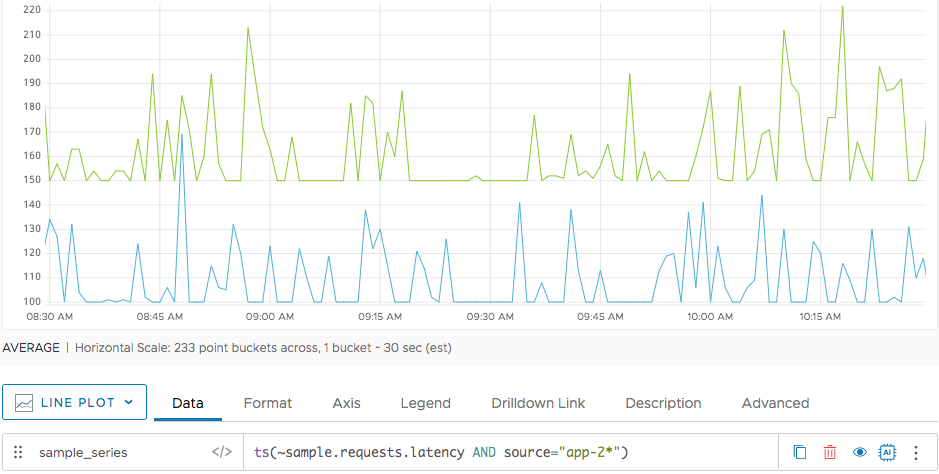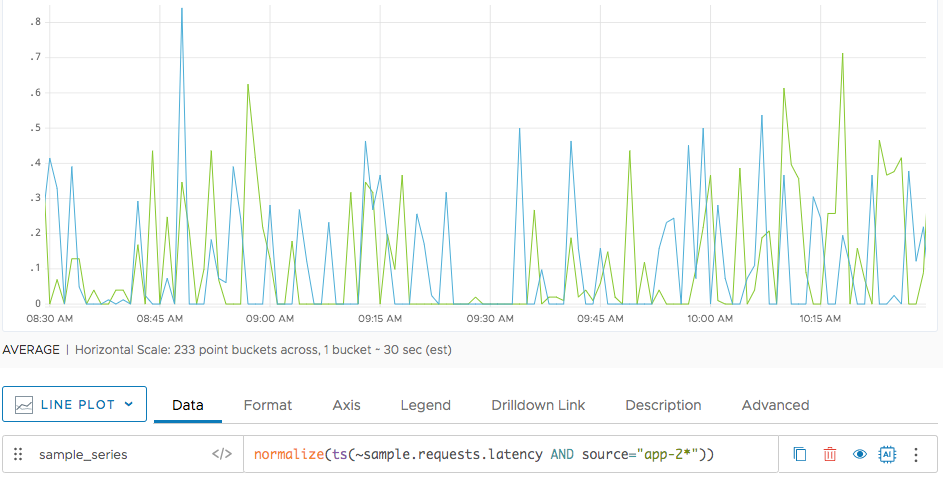Reference to the normalize() function

## Summary

``````normalize(<tsExpression>)
``````

Returns the time series described by the expression, scaled to fit between the values 0 and 1.0.

## Parameters

ParameterDescription
tsExpression Expression describing the time series you want to normalize.

## Description

The `normalize()` function scales the time series described by the expression, so that each series has a minimum value of 0 and maximum value of 1. `normalize()` returns a separate series of results for each time series.

Normalizing is useful if you want to see shape correlation between time series of very different scale. After normalizing, all the time series fit in the same vertical space.

## Examples

The following diagram shows the request latency for `app-2` and `app-20`.When you normalize the data, the metrics for both time series are mapped to values between 0 and 1.